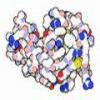#### You may also like### Mathematical Issues for Chemists

A brief outline of the mathematical issues faced by chemistry students.### Reaction Rates

Explore the possibilities for reaction rates versus concentrations with this non-linear differential equation### Mixed up Mixture

Can you fill in the mixed up numbers in this dilution calculation?

# Molecular Sequencer

##### Age 14 to 18 Challenge Level:

Pentanal is $C_5H_{10}O$
Hexanal is $C_6H_{12}O$

By looking at the sequence of molecular formulae, it can be seen that as the carbon chain increases by one, the number of hydrogens increases by 2, whereas the number of oxygens remains at one.

Therefore, the nth molecule is given by $C_nH_{2n}O$.

Note that in actuality the masses of the different isotopologues (i.e. molecules which differ only in their isotopic composition) of say $\text{CH}_4$ are slightly different. These differences may be noted by a very sensitive mass spectrometer.

Take for example: RMM $^{12}\text{CH}_3\text{D}$ = 12 + 3(1.007825) + 2.014102 = 17.037577 gmol$^{-1}$ RMM $^{13}\text{CH}_4$ = 13.00335 + 4(1.007825) = 17.03465 gmol$^{-1}$ where the calculation is limited by the degree of accuracy of the given data.

However in this question it is acceptable to take values to the nearest gmol$^{-1}$, giving roughly equal molecular masses for certain isotopes.

The lightest isotopologue is $^{12}C_n\ ^1H_{2n}\ ^{16}O$ which has a molecular mass of $14n +16$.

The heaviest isotopologue is $^{13}C_n\ ^2H_{2n}\ ^{18}O$ which has a molecular mass of $17n +18$.

Additionally, all intermediate masses are also possible.

To work out the final part of this problem involves recognising that the lightest general form of an aldehyde is $^{12}C_n\ ^1H_{2n}\ ^{16}O$, whereas the next lightest is given by $^{12}C_n\ ^1H_{2n}\ ^{17}O$ and $^{12}C_{n-1}\ ^{13}C\ ^1H_{2n}\ ^{16}O$ and $^{12}C_n\ ^1H_{2n-1}\ ^2H\ ^{16}O$.

Therefore, the probability of the lightest isotopologue must be equal to eight times the sum of the probabilities of the other three forms given:

$$\begin{matrix} (0.989)^n (0.9999)^{2n} (0.9976) \\ = 8[(0.989)^n (0.9999)^{2n} (0.0004) \\ + (0.989)^{n}\ ^{2n}C_1 (0.9999)^{2n-1} (0.0001)(0.9976) \\ + ^nC_1(0.989)^{n-1}(0.011)(0.9999)^{2n}(0.9976)] \end{matrix}$$

This equation is best solved using a spreadsheeting program, and inputting different values of $n$ until both sides of the equation are roughly equal. This reveals that $\mathbf{n=11}$ satisfies the equation.